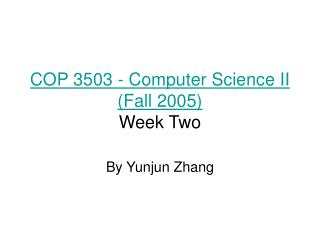DownloadDownload PresentationCOP 3503 - Computer Science II (Fall 2005) Week Two

# COP 3503 - Computer Science II (Fall 2005) Week Two

Télécharger la présentation## COP 3503 - Computer Science II (Fall 2005) Week Two

- - - - - - - - - - - - - - - - - - - - - - - - - - - E N D - - - - - - - - - - - - - - - - - - - - - - - - - - -
##### Presentation Transcript

1. COP 3503 - Computer Science II (Fall 2005)Week Two By Yunjun Zhang

2. Ch7 Exercises • 7.1 • What are the four fundamental rules of recursion?

3. Ch7 Exercises • 7.1 Answer • Base cases: You must always have some base cases, which can be solved without recursion. • Making progress: For cases that are to be solved recursively, the recursive call must be always be to a case that makes progress toward a base case. • Design rule. Assume that all the recursive calls work. • Compound interest rule. Never duplicate work by solving the same instance of a problem in separate recursive calls.

4. Ch7 Exercises • 7.2 • // Evaluate the sum of the first n integers public static long s( int n) { if( n==1) return 1; else return s(n-1)+n; } Modify the program above so that zero is returned for negative n. Make the minimum number of changes.

5. Ch7 Exercises • 7.2 Answer public static long s( int n) { if( n<=0) return 0; else return s(n-1)+n; }

6. Ch7 Exercises • 7.3 Modular Exponentiation Public static long power(long x, long n, long p) { if(n==0) return 1; long tmp=power((x*x)%p,n/2,p); if( n % 2 !=0) tmp=(tmp*x)%p; return tmp; } Given four alternatives of the red line, why they are wrong? • Long tmp=power(x*x,n/2,p); • Long tmp=power(power(x,2,p),n/2,p); • Long tmp=power(power(x,n/2,p),2,p); • Long tmp=power(x,n/2,p)*power(x,n/2,p)%p;

7. Ch7 Exercises • 7.3 Answer • Intermediate results may become too large • The recursion can only return when n = 0. This is infinite loop. • As above • Violate the compound interest rule and the result is O(N) multiplication

8. CH7 Exercises • 7.4 Show how the recursive calls are processed in the calculation 2^63 mod 37.

9. Ch7 Exercises • 7.4 Answer 2^63 mod 37 =(2(4^31 mod 37)) mod 37 4^31 mod 37 =(4(16^15 mod 37)) mod 37 16^15 mod 37=(16(256^7 mod 37))mod 37 =(16(34^7 mod 37)) mod 37 34^7 mod 37=(34(1156^3 mod 37))mod 37 =(34(9^3 mod 37)) mod 37 9^3 mod 37=(9(81^1 mod 37)) mod 37 =(9(7^1 mod 37)) mod 37 7^1 mod 37 = (7(49^0 mod 37)) mod 37 =7 9^3 mod 37 =(9*7) mod 37 =26 34^7 mod 37=(34*26) mod 37 =33 16^63 mod 37=(16*33) mod 37 =10 4^63 mod 37 = (4*10) mod 37= 3 2*63 mod 37 =(2*3) mod 37 =6

10. Ch7 Exercises • 7.5 // Return the greatest common divisor Public static long gcd( long a, long b) { if(b==0) return a; else return gcd( b, a%b); } Compute gcd(1995, 1492)

11. Ch7 Exercises • 7.5 Answer • Why it works • gcd(A,B)=gcd(A-B,B) • When A-B <B , switch the position • In order to reduce the number of subtractions, gcd(A,B)=gcd(B,A%B) • gcd(0,B)=B; • gcd(1995,1492) =gcd(1492,503) =gcd(503,486) =gcd(486, 17) =gcd(17,10) =gcd(10,7) =gcd(7,3) =gcd(3,1) =1

12. Ch7 Exercises • 7.6 skip

13. Ch7 Exercises • 7.7 Show that greedy change-making algorithm fails if 5-cent pieces are not part of US currency. change-making algorithm For a currency with coins C1,C2,…, CN(cents) what is the minimum number of coins needed to make K cents of change?

14. Ch7 Exercises • 7.7 Answer Example: 30 cents: by greedy, one quarter and five pennies. But the best result comes from 3 dimes

15. Ch7 Exercises • 7.8 The fibonacci numbers: F0,F1,F2,… are defined as follow: F0=0;F1=1; FN=F(N-1)+F(N-2); Prove by induction the formula

16. Ch7 Exercises • 7.8 Answer • Basis (N=0,N=1) • Assume the formula is true for all i<N, • Prove Fn=F(n-1)+F(n-2) • Details on board

17. Ch7 Exercises • 7.9 Prove the following identities relating to the Fibonacci numbers. F1+F2+F3+…+FN=F(n+2)-1

18. Ch7 Exercises • 7.10 Show that if A≡B(mod N), then for any C, D, and P, the following are true • A+C≡B+C(mod N) • AD≡BD(mod N) • A^P=B^P(mod N)

19. Ch7 Exercises • 7.10 Answer • A=a*N+ext,B=b*N+ext C=c*N+c’; A+C=(a+c)N+ext+c’; B+C=(b+c)N+ext+c’; (b) A=a*N+ext,B=b*N+ext AD=a*D*N+ext*D; BD=b*D*N+ext*D; • A^P=(a*N+ext)^p=ext^p+poly1(N) B^P=(b*N+ext)^p=ext^p+poly2(N)

20. Ch7 Exercises • 7.11 Prove that if A>=B, then A mod B < A/2.

21. Ch7 Exercises • 7.11 Answer Proof: if B<=A/2 A mod B <A/2 because the remainder should smaller than B. otherwise, the remainder is A-B<A/2